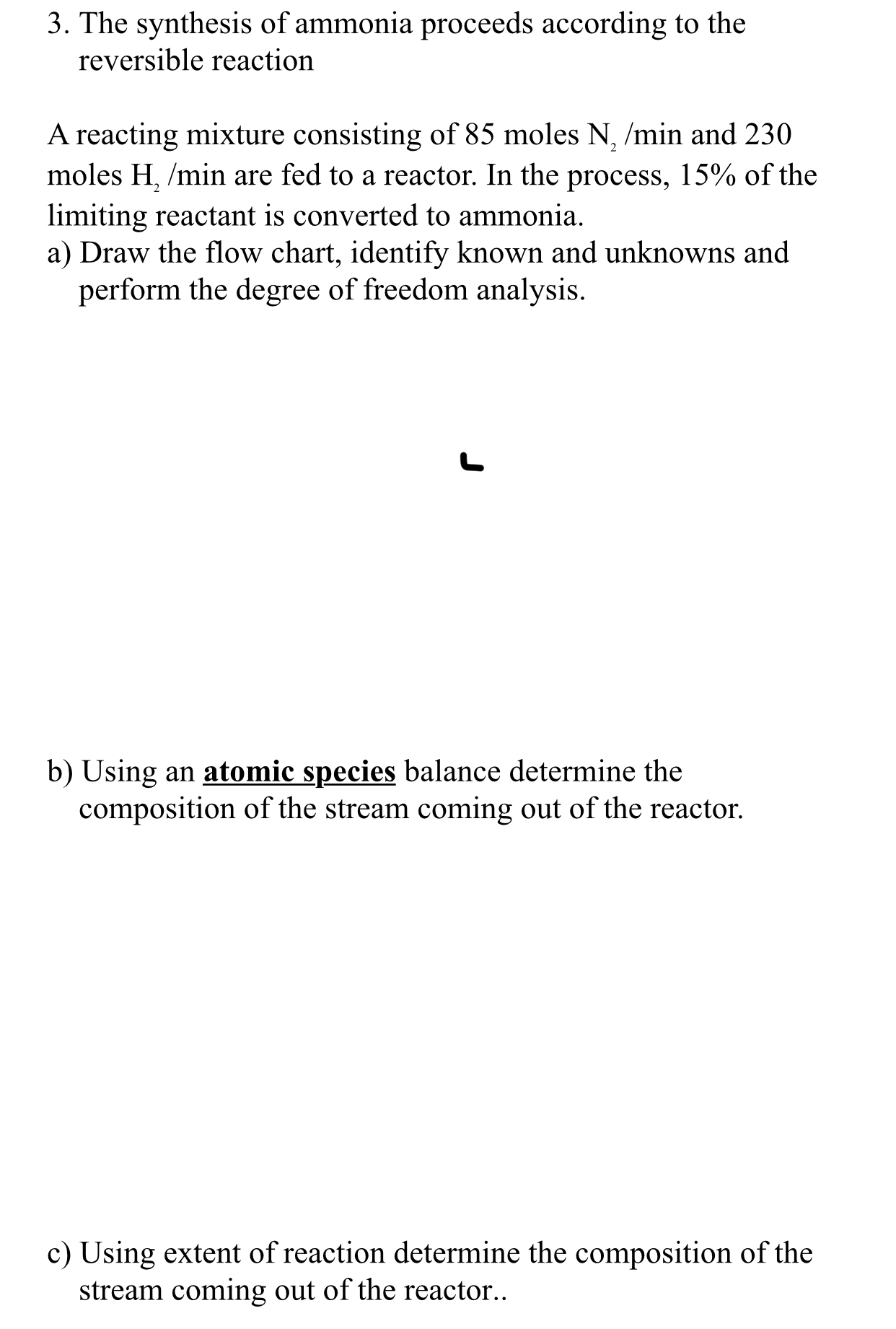# 3. The synthesis of ammonia proceeds according to thereversible reactionA reacting mixture consisting of 85 moles N, /min and 230moles H. min are fed to a reactor. In the process. 15% of thelimiting reactant is converted to ammoniaa) Draw the flow chart, identify known and unknowns andperform the degree of freedom analysisb) Using an atomic species balance determine thecomposition of the stream coming out of the reactorc) Using extent of reaction determine the composition of thestream coming out of the reactor.

Question
93 views

Inhelp_outlineImage Transcriptionclose3. The synthesis of ammonia proceeds according to the reversible reaction A reacting mixture consisting of 85 moles N, /min and 230 moles H. min are fed to a reactor. In the process. 15% of the limiting reactant is converted to ammonia a) Draw the flow chart, identify known and unknowns and perform the degree of freedom analysis b) Using an atomic species balance determine the composition of the stream coming out of the reactor c) Using extent of reaction determine the composition of the stream coming out of the reactor. fullscreen
check_circle

star
star
star
star
star
1 Rating
Step 1

Part (a)

The reversible reaction for the formation of ammonia from nitrogen and hydrogen is:

N2 + 3H2 ↔ 2NH3

From the stoichiometry of the above reaction, 1 mol of N2 reacts with 3 mol of H2. For 85 mol of N2/min, 255 mol H2/min is required. But, only 230 mol H2/min is given so, hydrogen (H2) is the limiting reactants and 15% of H2 is reacted to produce ammonia.

The flowchart of the given process is drawn below.

Step 2

The formula used to calculate degree of freedom (DF) is written below.

There are total 3 unknown variables present. These are, 1, 2, and 3.

There are 3 independent equations, 2 equation for material balance and 1 equation for conversion condition.

Therefore, degree of freedom for the given process is 0.

Step 3

Part (b)

Take atomic species for the material balance and calculate the composition of the product stream.

Apply material balance for nitrogen (N) and label the equation as (1).

Apply material balan...

### Want to see the full answer?

See Solution

#### Want to see this answer and more?

Solutions are written by subject experts who are available 24/7. Questions are typically answered within 1 hour.*

See Solution
*Response times may vary by subject and question.
Tagged in

### Chemical Engineering